﻿ Neural networks tutorial: Testing analysis | Neural Designer

# 6. Testing analysisThe purpose of testing is to compare the outputs from the neural network against targets in an independent set (the testing instances). Note that the testing methods are subject to the project type (approximation or classification).

If all the testing metrics are considered ok, the neural network can move to the so-called deployment phase. Note also that the results of testing depend very much on the problem at hand, and some numbers might be right for one application but bad for another.

The validation methods that need to be used depending on the application type:

## 6.1. Approximation testing methods

The most used testing methods in approximation applications are the following:

### Testing errors

To test a neural network, you may use any of the errors described in the loss index page as metrics.

The most critical errors for measuring the accuracy of a neural network are:

• Sum squared error.
• Mean squared error.
• Root mean squared error.
• Root mean squared logarithmic error.
• Normalized squared error.
• Minkowski error.
All those errors are measured on the testing instances of the data set.

### Errors statistics

The errors statistics measure the minimums, maximums, means, and standard deviations of the errors between the neural network and the testing instances in the data set.

The following table contains the basic statistics on the absolute and percentage error data when predicting the electricity generated by a combined cycle power plant.
Minimum Maximum Mean Deviation
Absolute error 0.03 18.13 4.18 5.27
Percentage error 0.05% 29.46% 6.79% 8.57%

### Errors histogram

Error histograms show how the errors from the neural network on the testing instances are distributed. In general, a normal distribution centered at 0 for each output variable is expected here.

The next figure illustrates the histogram of errors made by a neural network when predicting sailing yachts' residuary resistance. Here the number of bins is 10.### Maximal errors

It is very useful to see which testing instances provide the maximum errors, and alert for deficiencies in the model.

The following table illustrates the maximal errors of a neural network for modeling the adhesive strength of nanoparticles.

Rank Index Error Data
1 30 9.146 shear_rate: 75
particle_diameter: 4.89
2 35 9.121 shear_rate: 75
particle_diameter: 6.59
3 11 6.851 shear_rate: 50
particle_diameter: 4.89

As we can see, particles with a shear rate of 75 seem to yield the most significant errors.

### Linear regression analysis

Linear regression analysis is the most standard method to test the performance of a model in approximation applications.

Here the neural network outputs and the corresponding data set targets for the testing instances are plotted.

This analysis leads to 3 parameters for each output variable:

The first two parameters, a and b, correspond to the y-intercept and the slope of the best linear regression relating to scaled outputs and targets. The third parameter, R2, is the correlation coefficient between the scaled outputs and the targets.

For a perfect fit (outputs equal to targets), the slope would be 1, and the y-intercept would be 0. If the correlation coefficient is equal to 1, then there is a perfect correlation between the outputs from the neural network and the targets in the testing subset.

The following figure is a plot of the linear regression analysis for predicting the noise generated by airfoil blades. As we can see, all the predicted values are very similar to the real ones.The parameters led by this analysis are correlation = 0.952, intercept = 13.9 and slope = 0.89. From that, the correlation coefficient is the most important parameter. As this value is very close to 1, we can say that the neural network predicts the noise very well.

## 6.2. Classification testing methods

The most common testing methods in classification applications are the following:

### Confusion matrix

In the confusion matrix, the rows represent the target classes in the data set and the columns the corresponding output classes from the neural network

The diagonal cells in each table show the number of correctly classified cases, and the off-diagonal cells show the misclassified cases.

For binary classification, positive means identified, and negative means rejected. Therefore, 4 different cases are possible:

• True positive (TP): correctly identified.
• False positive (FP): incorrectly identified.
• True negative (TN): correctly rejected.
• False negative (FN): incorrectly rejected.

Note that the output from the neural network is, in general, a probability. Therefore, the decision threshold determines the classification. The default decision threshold is 0.5. The output above is classified as positive, and the output below is classified as negative.

For the case of two classes, the confusion matrix takes the following form:

Predicted positive Predicted negative
Real positive true_positives false_negatives
Real negative false_positives true_negatives

The following example is the confusion matrix for a neural network that assesses the risk of credit card clients' default.

Predicted positive Predicted negative
Real positive 745 (12.4%) 535 (8.92%)
Real negative 893 (14.9%) 3287 (63.8%)

For multiple classification, the confusion matrix can be represented as follows:

Predicted class 1       ···       Predicted class N
Real class 1 # # #
··· # # #
Real class N # # #

The following example is the confusion matrix for the classification of iris flowers from sepal and petal dimensions.

Predicted setosa Predicted versicolor Predicted virginica
Real setosa 10 (33.3%) 0 0
Real versicolor 0 11 (36.7%) 0
Real virginica 0 1 (3.33%) 8 (26.7%)

As we can see, all testing instances are correctly classified, except one, which is an iris Virginica that has been classified as an iris Versicolor.

### Binary classification tests

For binary classification, there are a set of standard parameters for testing the performance of a neural network. These parameters are derived from the confusion matrix:

• Classification accuracy: Ratio of instances correctly classified. An accuracy of 100% means that the measured values are the same as the given values. $$classification\_accuracy = \frac{true\_positives+true\_negatives}{total\_instances}$$
• Error rate: Ratio of instances misclassified, $$error\_rate = \frac{false\_positives+false\_negatives}{total\_instances}$$
• Sensitivity, or true positive rate: Proportion of actual positive which are predicted positive, $$sensitivity = \frac{true\_positives}{positive\_instances}$$
• Specificity, or true negative rate: Proportion of actual negative which are predicted negative, $$specificity = \frac{true\_negatives}{negative\_instances}$$
• Positive likelihood: Likelihood that a predicted positive is an actual positive, $$positive\_likelihood = \frac{sensitivity}{1-specificity}$$
• Negative likelihood: Likelihood that a predicted negative is an actual negative, $$negative\_likelihood = \frac{1-sensitivity}{specificity}$$

The following lists the binary classification tests corresponding to the confusion matrix for assessing the risk of default of credit card clients that we saw above:

• Classification accuracy: 0.762
• Error rate: 0.238
• Sensitivity: 0.582
• Specificity: 0.810
• Positive likelihood: 3.076
• negative likelihood: 1.939

From those parameters, we can see that the model is classifying very well the negatives (specificity = 81.0%), but it has not such a high precision for the positives (sensitivity = 58.2%).

### ROC curve

A standard method for testing a neural network in binary classification applications is to plot a ROC (Receiver Operating Characteristic) curve.

The ROC curve plots false positives rate (or 1 - specificity) on the X-axis, and true negatives rate (or sensitivity) on the Y-axis for different decision threshold values.

An example of the ROC curve for diagnosing breast cancer from fine-needle aspirate images is the following.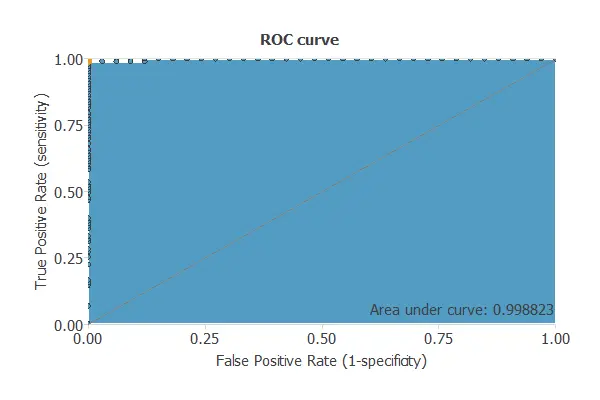The capacity of discrimination is measured by calculating the area under the curve (AUC). A random classifier would give AUC = 0.5 and a perfect classifier AUC = 1.

For the above example, the area under the curve is AUC = 0.994. That is an excellent value, indicating that the model is predicting almost perfectly.

### Cumulative gain

The cumulative gain analysis is a visual aid that shows the advantage of using a predictive model instead of randomness.

It consists of two lines:

• The baseline represents the results that would be obtained without using a model.
• The positive cumulative gain shows in the y-axis the percentage of positive instances found against the percentage of the population, which is represented in the x-axis.
• The negative cumulative gain shows the percentage of the negative instances found against the percentage of the population.

The next chart shows the results of the analysis for a model that predicts if a bank client is likely to churn. The blue line represents the positive cumulative gain, the red line represents the negative cumulative gain, and the grey line represents the cumulative gain for a random classifier.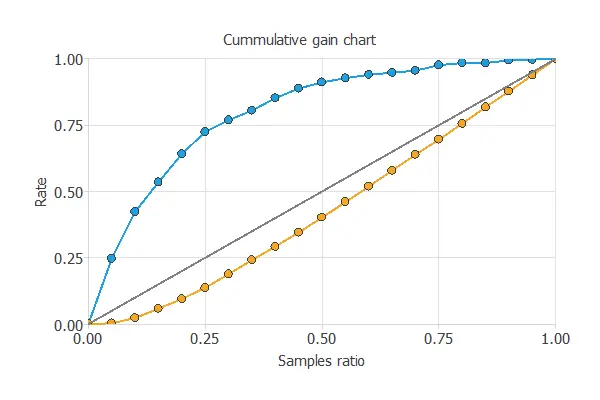In this case, by using the model, we see that by analyzing the 50% of the clients with the higher probability of churn, we would reach 80% of clients going to leave the bank.

### Lift chart

This method provides a visual aid to evaluate a predictive model loss. It consists of a lift curve and a baseline. The lift curve represents the ratio between the positive events using a model and without using it. Baseline represents randomness, i.e., not using a model.

The x-axis displays the percentage of instances studied. The y-axis displays the ratio between the results predicted by the model and the results without the model. Below is depicted the lift chart of a model that predicts if a person is going to donate blood.To explain the usefulness of this chart, let us suppose that we are trying to find the positive instances of a data set. If we try to find them randomly, after studying 50% of the instances, we discover 50% of the positive instances.

Now, let suppose that we first study those instances that are more likely to be positive according to the score that they have received from the classifier. After studying 50% of them, we find 80% of positive instances.

Therefore, by using the model, after studying 50% of the instances, we would discover 1.6% more positive instances than by looking for them randomly. This advantage is what is represented in the lift chart.

### Positives and negatives rates

Positive rates tell us how the selection of positives changes by using a classification model.

In marketing applications, the positive rate is called the conversion rate. Indeed, it depicts the number of clients that respond positively to our campaign out of the number of contacted clients.

The next chart shows two rates. The first column represents the data set rates, while the second one represents the ratios for the predicted positives of the model.### Profit chart

This testing method shows the difference in profits from randomness and those using the model depending on the instance ratio.

In this chart, three lines are represented. The grey one represents the profits from randomly choosing the instances. The blue line is the evolution of the profits when using the model. Finally, the black one separates the benefits from the losses of the process. The chart below represents the profit chart of a model that detects forged banknotes.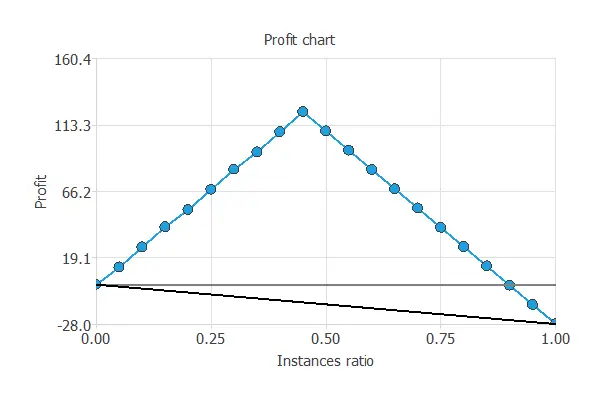The values of the previous plot are displayed below.

• Unitary cost: 1
• Unitary income: 2
• Maximum profit: 122.7
• Instance ratio: 0.45

The maximum profit is the value of the greatest benefit obtained with the model, 122.7%, and the instance ratio is the percentage of instances used to obtain that benefit, 45%.

### Misclassified instances

When performing a classification problem, it is essential to know which instances have been misclassified. This method shows which actual positive instances are predicted as negative (false negatives) and which actual negative instances are predicted as positive (false positives).

This information is displayed in a table format, identifying each instance with an Instance ID, and showing its corresponding data, composed of its values of the model variables. Two tables are displayed, one for the positive instances predicted as negative and the other for the negative instances predicted as positive.

## 6.3. Forecasting testing methods

All testing methods used for approximation models are valid for forecasting models.

But there are specific testing methods for prediction models. Some of them are the following:

### Outputs plot

A visual method for testing a forecasting model is to plot the time series data and their corresponding predictions against time to see the differences.

The next chart shows the one step ahead of average temperature in a city (blue) and their corresponding predictions (orange).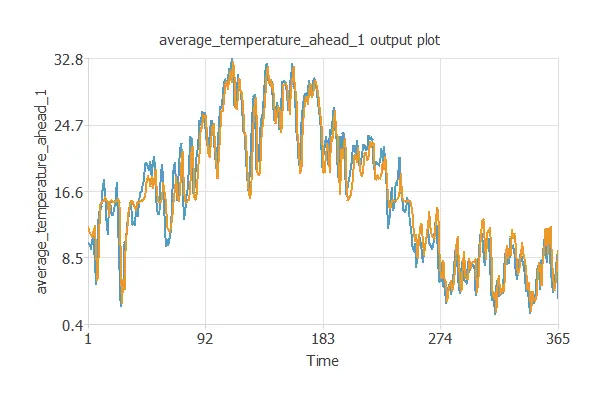### Error autocorrelation

The error autocorrelation function describes how prediction errors are correlated in time.

The following chart depicts the error autocorrelation for the 1-day temperature forecast in a city. The abscissa represents the lags and the ordinate their corresponding correlation value.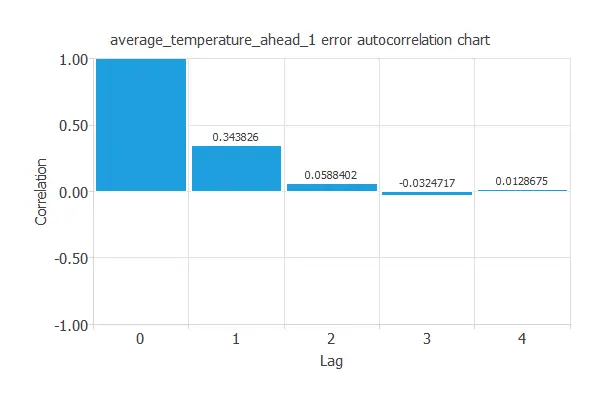As we can see, the lag 1 correlation is positive (0.344). That means that if our prediction error was big yesterday, we might expect a big prediction error today.

### Error cross-correlation

This task calculates the correlation between the inputs and the prediction error.

The following chart depicts the error cross-correlation for the 1-day temperature forecast in a city. The abscissa represents the lags and the ordinate their corresponding correlation value.As we can see, although the cross-correlations have small values, they are positive. That means that, in general, the bigger the prediction value, the bigger error we can make.